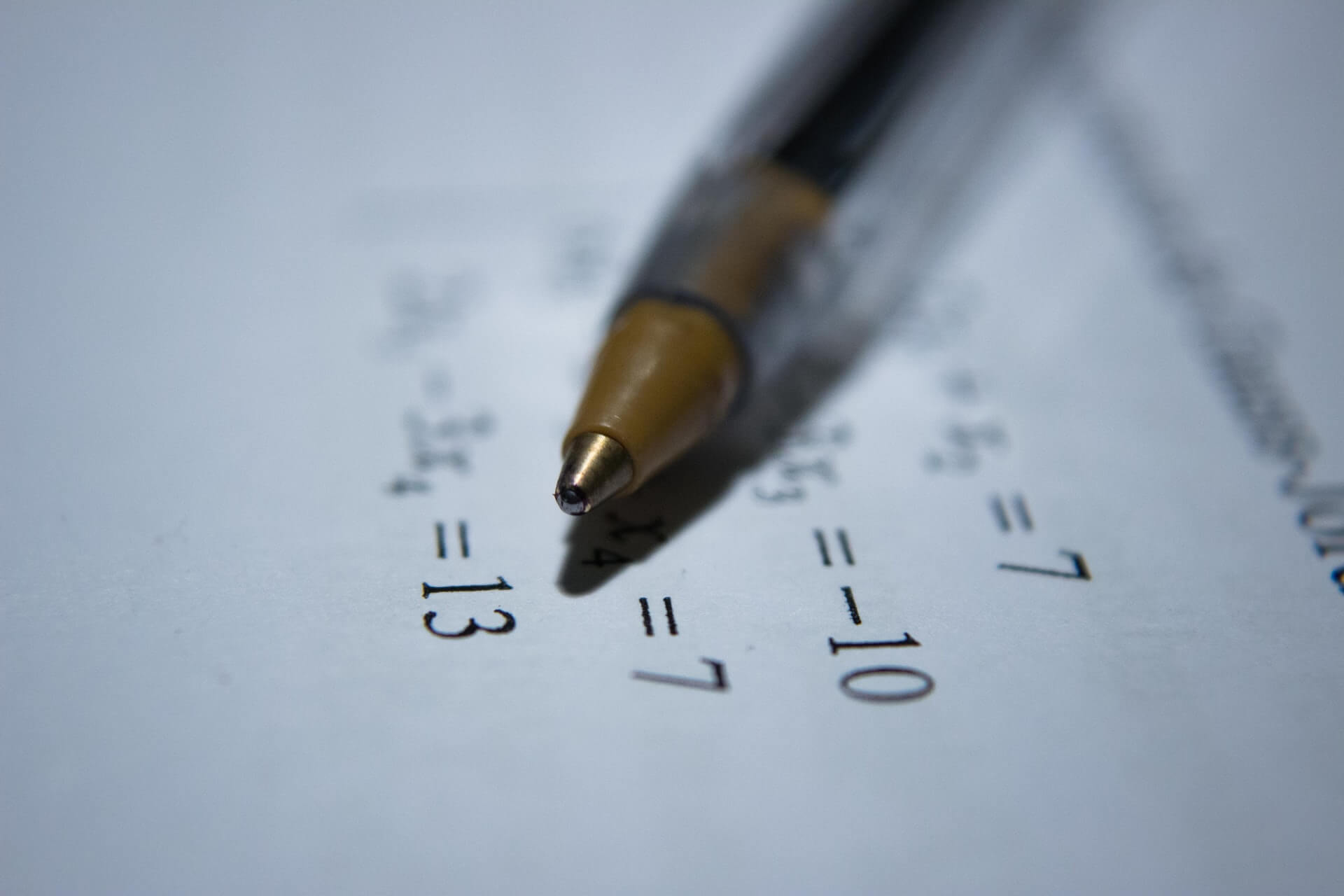# Learn Math Fast with these tips and tricks

October 11, 2022Math issues can be intimidating to many students. This is especially true if the problems contain large numbers. However, it can get simpler with the correct instruction, practice, and dedication. Students who master rapid math tricks do better in challenging courses, have more math confidence, and have better arithmetic skills and understanding.

It could take more work for people who desire to improve their math skills. Here are a few suggestions to learn math fast.

Math relies on the fundamentals. For instance, you must first grasp concepts like addition, subtraction, division, and multiplication. Then, as your understanding grows, you can master more challenging math concepts like algebra, geometry, and calculus.

Algebra can get difficult if you don’t know how to do addition or division. Additionally, you can’t be good at calculus without a strong background in algebra and trigonometry. Understanding the fundamentals is crucial to building on them.

## Set Objectives

Once you’ve learned math fundamentals, you can decide what you want to focus on. For example, there is no need to concentrate on geometry if your goal is to excel at algebra. Once you know the type of math you must learn, you may choose the quickest route and focus your efforts on becoming an expert in those areas.

Imagine you are trying to solve a math issue, but you keep coming up with the wrong solutions. You must be making faults somewhere.

It’s essential to pay attention to your errors as you work through a math issue. So, recognize your mistakes.

## Practice

The best method to get better at anything is to practice, regardless of the subject or discipline. Online and booklets both have practice issues. Additionally, complete all your assignments if you’re in school and taking a math class. Without a doubt, your teacher will be a valuable resource for providing you with additional practice problems.

## Why Is Math So Hard For Some Students?

Math requires much more practice than other courses because it frequently involves multiple steps to solve issues. This is the reason most students find math harder than other subjects.

Some students get bored when they have to do repetitive things, which may cause them to lose patience. Make math less challenging with these simple tips and tricks.

## Tips And Tricks To Learn Math Fast

There is no one way to become proficient in math because the subject is so broad. There are. However, tried-and-true best practices can assist you in getting better with each stage of your path.

By using these cool math tricks, students and teachers can develop their mathematical abilities, feel more confident in their understanding of the subject, and become less apprehensive when dealing with numbers in the future.

## Subtracting from 1,000

To remove a large number from 1,000, follow this simple formula: Subtract all numbers from 9 except for the last one, then subtract the final number from 10, and you’ll get your answer.

Let’s take a look at an example: $$1000 – 446$$

• Subtract 4 from 9 = 5
• Subtract 4 from 9 = 5
• Finally, subtract 6 from 10 = 4

## Multiplying five times a number

There is a simple math trick to determining the result when multiplying five by an even number.

For example, $$5 × 10 = ?$$

• Take the number multiplied by five and cut it in half. This makes the number 10 become the number 5.
• Add a zero to the number you obtained to find the result. Here, the answer is 50.

$$5 × 10 = 50$$

The formula is slightly different when multiplying an odd integer by 5.

For instance, let’s take $$5 × 7$$.

• Subtract one from the number multiplied by 5. Here, the number 7 becomes the number 6.
• Halve the number 6; you’ll get the number 3. Make five the last digit. The number we get here is 35, which is also the answer.

$$5 × 7 = 35$$

## Finding percentages

Finding a percentage of a number can be a little tough, but approaching it the right way becomes much simpler to comprehend. For instance, use the following strategy to determine what 5% of 345 is:

• Move the decimal point over by one place. 345 becomes 34.5.
• Divide 34.5 by the number 2. The answer is 17.25. Which is the answer to the original equation.

## Multiplying numbers ending with 0

Multiplying numbers with a zero at the end is easy. The other numbers are multiplied together, and the zeros are added at the end. For instance, think about the following:

$$300 × 600$$

Step 1: Multiply the three times the 6

$$3 × 6 = 18$$

Step 2: Put all four of the zeros after the 18

180,000

$$300 × 600 = 180000$$

## Final Words

Learning takes time, and each person has effective learning strategies, regardless of the subject you’re trying to master. But having a strong understanding of the fundamentals is the primary condition for learning arithmetic quickly. Then, you can work on expanding upon each topic with practice, further assistance, and helpful hints and recommendations.